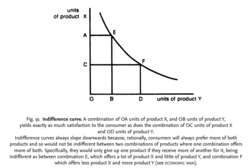# indifference curve

(redirected from Utility curve)

## Indifference curve

The expression in a graph of a utility function, where the horizontal axis measures risk and the vertical axis measures expected return. The curve connects all portfolios with the same utility.

## Indifference Curve

A curve on a graph where the x-axis represents a quantity of one good and the y-axis represents a quantity of a second good where the curve represents the universe of quantities with the same utility for a rational investor. The indifference curve is convex, or roughly U-shaped.Fig. 91 Indifference curve. A combination of OA units of product X, and OB units of product Y, yields exactly as much satisfaction to the consumer as does the combination of OC units of product X and OD units of product Y.

Indifference curves always slope downwards because, rationally, consumers will always prefer more of both products and so would not be indifferent between two combinations of products where one combination offers more of both. Specifically, they would only give up one product if they receive more of another for it, being indifferent as between combination E, which offers a lot of product X and little of product Y, and combination F which offers less product X and more product Y (see ECONOMIC MAN).

## indifference curve

a curve showing alternative combinations of two products, each of which gives the same UTILITY, or satisfaction. See Fig. 91 . Indifference curves are used (along with BUDGET LINES) to determine a consumer's equilibrium purchases of two products and to analyse the effect of changes in the relative prices of these two products upon quantities demanded (see PRICE EFFECT). See CONSUMER EQUILIBRIUM, INDIFFERENCE MAP.
References in periodicals archive ?
The "Critical Risk-Aversion Level" section focuses on the critical value of risk aversion at which 1-year expected utility curve inverts and relates this value to the parameters characterizing the funding ratio process.
So, the utility curve [U.sub.(+)] along the new curve [U.sup.1.sub.(+)] entered a new round of industrial life cycle.
In (5), [[delta].sub.HEU] and [[delta].sub.MEU] represent the steepness of the utility curve for HEU and MEU, while [R.sub.HEU] and [R.sub.NLU] determine the center of the utility curves for HEU and MEU Note that
Using these reference points, where the worst value is assigned 0 points, and the best assigned 100 points, a "utility curve" can be created to describe the value space of that particular requirement.
Also, it can be noticed that all the upper set of utility curve are convex that illustrates the property of quasi-concavity of utility function.
Based on these five sets of values, we can further estimate the utility curve of the average delay and use statistical software to obtain a utility function that approximates the curve.
He contends that the representative agent's utility is influenced by real consumption and real money balances, adding that the utility curve is strictly concave, twice differentiable and that both real consumption and real money balances are not inferior either as a good in the case of real consumption or as a service in the case of real money balances.
Most famously, Friedman and Savage (1948) motivated their double inflection, "wiggly" utility curve with a loose reference to implicit wealth dynamics that makes it difficult for individuals to move to higher socioeconomic classes and hence risk seeking when upside payoffs allow them to move to a higher class.
All parties involved with the utility curve will be glad you did.
A mathematician might draw a utility curve to find the point at which it no longer makes sense to shell out for a service; everyone else just goes on their gut feeling.
That is, the expected utility curve passing through the point [l.sup.RS] is obviously crossed by the pooling premium line (1 - [P.sub.A])/[P.sub.A].

Site: Follow: Share:
Open / Close